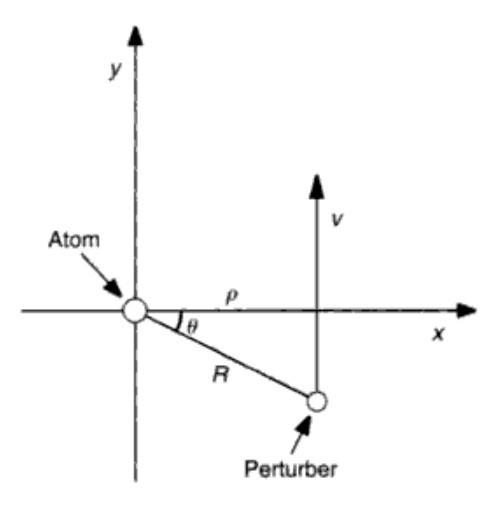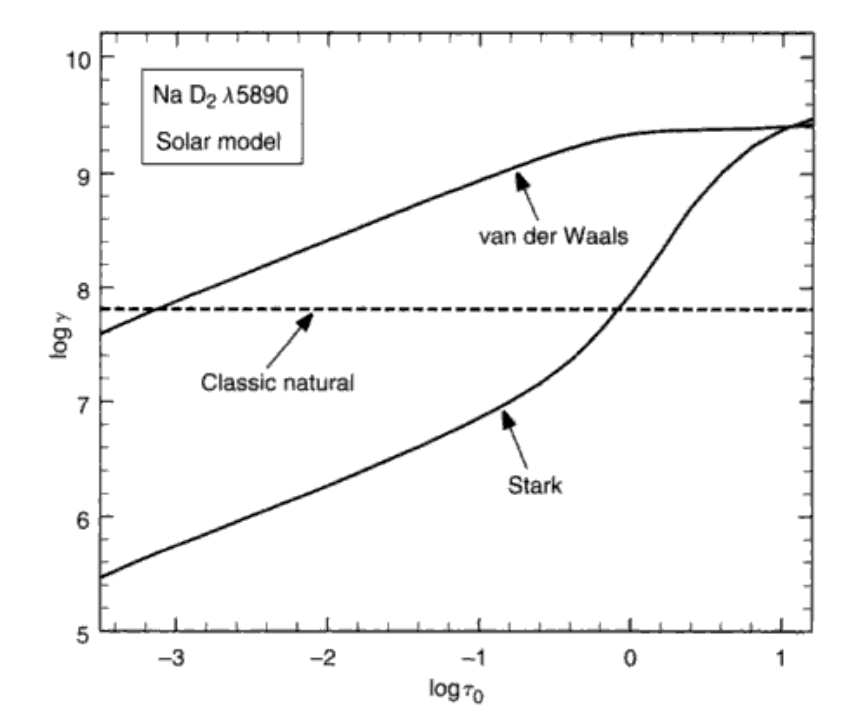# OASP笔记 - 11

## 谱线吸收系数

Posted by mingjie on May 16, 2018

### 自然致宽

$N$为电偶极子数密度、$q$为电偶极子电量（之后一般会换成$e$）、$z$为电偶极子长度。把电偶极子看成谐振子($\frac{d^2 z}{dt^2}$)，加上驱动力($\omega_0^2 z$)和阻尼($\gamma \frac{dz}{dt}$)，就有振动方程：

$x = 0$时方程有解为$z = z_0 e^{i\omega t}$，所以$\dot{z} = i\omega z, \ddot{z} = -\omega^2 z$，方程变为：

$\epsilon$很接近$\epsilon_0$，所以有泰勒展开：

$(6.8)$$B_{lu}$换成$A_{lu}$，有

### 压力致宽

$n$ 种类 影响的谱线 粒子
2 线性斯塔克效应 氢线 光子、电子
3 共振致宽   同种原子
4 二次斯塔克效应 高温恒星中的多数谱线 离子、电子
6 范德瓦尔斯效应 低温恒星中的多数谱线 中性氢

$C_n$需要被测出来。

#### 压力致宽的阻尼系数原子受影响的示意图

n $\int_{-\pi/2}^{\pi/2} \cos^{n-2}{\theta} d\theta$
2 $\pi$
3 $2$
4 $\pi/2$
5 $4/3$
6 $3\pi/8$

#### 两个例子Na I D2线的damping constent

### 热运动致宽

$(11.1)$

$v_0^2 = 2kT/m$，那么就有

### 将轮廓合起来

$a = 0$时，Hjerting函数就变回高斯了。我们也可以用Voigt函数来表示Hjerting函数，$V(u,a) = H(u,a)/(\pi^{1/2}\Delta\nu_\mathrm{D}$)。

### 差不多就是这样